GeeksforGeeks App
Open AppBrowser
Continue

Consider a set A and U denotes the universal set then complement of set A can be found by

`Ac =U-A.`

But, a graph G(v, e) is a set of vertices and edges, is it possible to find the Complement of a graph? This question is answered below.

### Complement of Graph

The complement of a graph G is a graph G’ on the same set of vertices as of G such that there will be an edge between two vertices (v, e) in G’, if and only if there is no edge in between (v, e) in G.

Complement of graph G(v, e) is denoted by G'(v, e’).

Note: The number of vertices remains unchanged in the complement of the graph.

Example: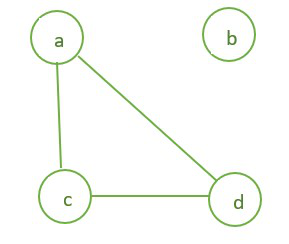Graph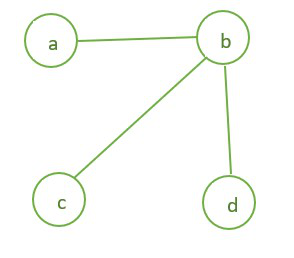Complemented Graph

```In the above example in graph G there is a edge between (a, d),(a, c),(a, d).
Complement of Graph G is G' having edges between (a, b),(b, c),(b, d). ```

#### Properties of Complemented Graph

1. If E be the set of edges of graph G’ then E(G’)={ (u, v) | (u, v) ∉ E(G) }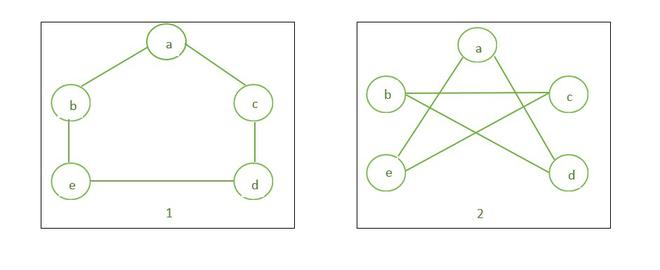Graph and its Complement

2. Union of graph G and its complement G’ will give a complete graph(Kn).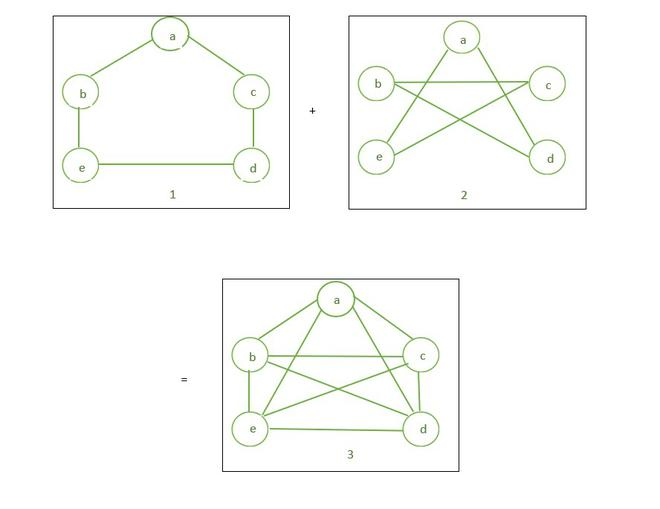Union of Graph and Complemented Graph

3. The intersection of two complement graphs has no edges, also known as null graph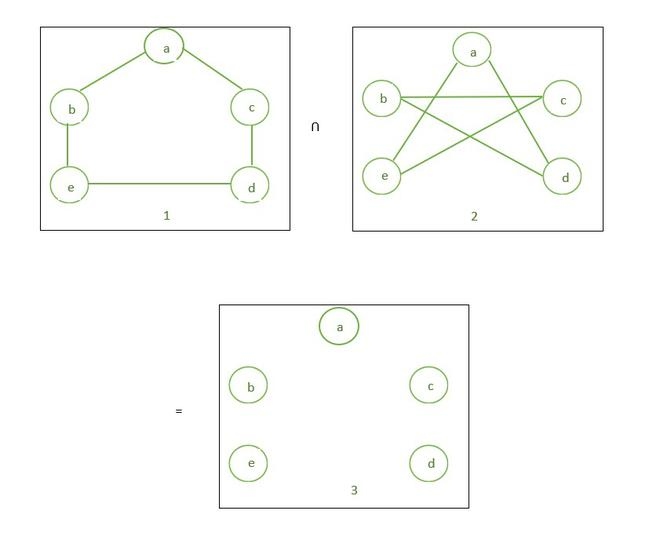The intersection of the graph and complemented graph

4. If G is a disconnected graph then its complement G’ would be a connected graph.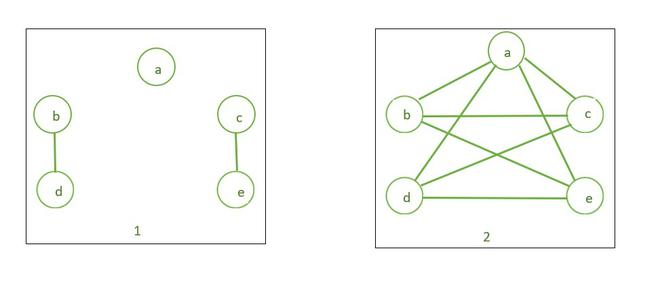The complement of a Disconnected graph is connected

5. Order of a Graph and its Complement are Same. The order of the graph is the number of vertices in it.

Example:

Order of a graph G on a set of vertices is given by G={a, b, c, d, e} is number of vertices in the graph G i.e., 5.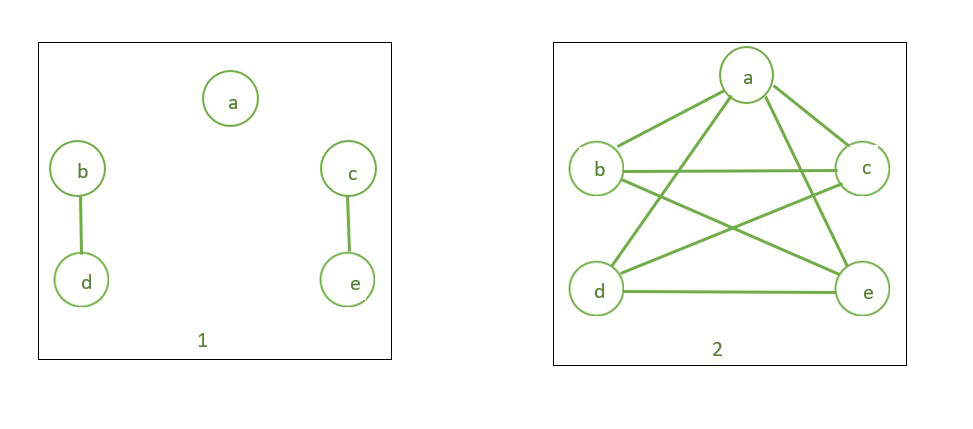The order of  Graph 1 and its complement 2 is the same.

6. Size of a Graph and its complement cannot be the same. The size of a graph is the number of edges in it.

Example:

Size of a graph G on the set of edges is G= {(b, d), (c, e) } is the number of edges in the graph i.e., 2.The size of Graph 1 is 2 and the Size of its Complement Graph 2 is 8

Note:

1. If G be a graph with edges E and Kn denoting the complete graph, then the complement of graph G can be given by

`E(G') = E(Kn)-E(G).`

2. The sum of the Edges of a Complement graph and the main graph is equal to the number of edges in a complete graph, n is the number of vertices.

`E(G')+E(G) = E(Kn) = n(n-1)÷2.`

#### Practice Questions:

Question 1. Consider a simple graph G, where E denotes the edges and V denotes the vertices |E(G)|= 30, |E(G’)|= 36. Find |V(G)|=?

Solution

```        We know,
E(G')+E(G)=E(Kn)=n(n-1)÷2.
⇒ 36+30=n(n-1)÷2
⇒ 66=n(n-1)÷2
⇒ 66×2=n2-n
⇒ n2-n-132=0
⇒ n2-12n+11n-132=0
⇒ n(n-12)+11(n-12)=0
⇒ (n-12)(n+11)=0
Therefore, n=12 and n=-11.
Since vertices cannot be negative
n=12.```

Question 2. Consider a simple graph G, where E denotes the edges and V denotes the vertices |E(G)|= 12, |V(G)|= 8. Find the number of edges in complement graph |E(G’)|= ?.

Solution

```       We know,
E(G')+E(G)=E(Kn)=n(n-1)÷2.
⇒ E(G') + 12 =8(8-1)÷2      [here n denotes number of vertices, i.e. given 8]
⇒ E(G')+12 = 8(7)÷2
⇒ E(G')+12= 4×7
⇒ E(G')+12=28
⇒ E(G')=28-12
⇒ E(G')=16.```
My Personal Notes arrow_drop_up